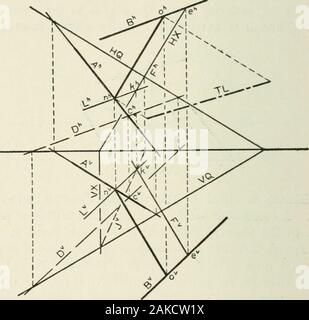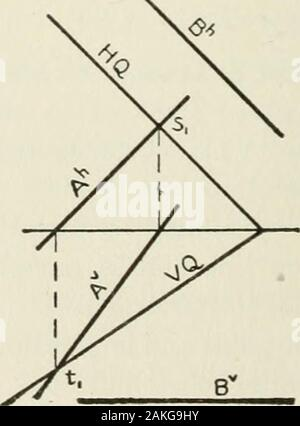RM2AKCW1XDescriptive geometry . is also in Q, and since L is not parallel to A becauseB is not, L and A will intersect in a point, n. Let the per-pendicular ek come to rest when point k coincides with point n.The line will then be in the position on, intersecting both Aand B and perpendicular to each. Hence on is the commonperpendicular required. The actual distance between thelines A mid Ji may ho found by measuring the length ofeither ek or on. 156 DESCRIPTIVE GEOMETRY [XVI, § 144 Construction (Fig. 237). Let A and B be the given lines.Through A pass the plane Q, parallel to B (Prob. 7, § 107).To doRM2AKG9HYDescriptive geometry . Fig. 143. Construction (Fig. 143). Let it be required to pass the planethrough the line A. Assume any point con J; through c drawthe line D parallel to B. Pass the required plane Q throughthe lines A and D (Prob. 6, § 106).. Fig. 144. X, § 107] PARALLEL LINES AND PLANES 85 Special Case I. Suppose the second line is parallel to IIor V; then no auxiliary line is needed. Thus, in Fig. 144, letus find the plane which contains the line A and is parallel toB. The .ff-trace of any plane which is parallel to B must beparallel to Bh (§ 104). Hence, find the traces of A. DrawHQ th